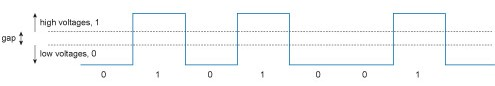Science, Maths & Technology

### Become an OU studentAn introduction to computers and computer systems

Start this free course now. Just create an account and sign in. Enrol and complete the course for a free statement of participation or digital badge if available.

# 4 An alternative to binary?

Some very early computers, such as the ENIAC you saw in Session 1, tried to represent data using our usual base-10 system. So 0 volts was used to represent the digit 0, 1 volt to represent the digit 1, and so on, all the way up to 9 volts to represent the digit 9. However, having a range of different values caused problems: voltage is not steady in a circuit, it varies as the circuit is switched on or off, and it also varies as the electricity flows through components. To cope with this, a lot of circuitry was needed just to distinguish between the different voltages, which took up a lot of space and generated a lot of heat.

The advantage of representing data in binary is that only two ranges of voltage need to be detected. The actual voltage values are defined in the specification of the electronic transistors used in a processor. In a particular transistor, any voltage between, say, 0 and 1.3 V (‘low’ voltages) might be interpreted as the digit 0, and any and any voltage above 1.7 V (‘high’ voltages) might be interpreted as the digit 1. In this case, the circuit would be designed to prevent voltages between 1.3 and 1.7 V. This gap means that if there are any small random dips or increases in the voltage (called noise), the two binary digits will still be distinguishable.Figure 1 Representing 0s and 1s using high and low voltages.

This simple to implement and efficient method of representing binary data with high- and low-voltages is the basis for contemporary processors. However, we don’t think in binary digits grouped into bytes. In the next section you will see how bytes can be rendered as something we humans understand.